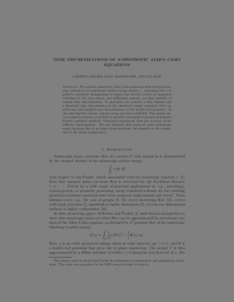Repository: Freie Universität Berlin, Math Department

# Time discretizations of anisotropic Allen-Cahn equations

Gräser, C. and Kornhuber, R. and Sack, U. (2013) Time discretizations of anisotropic Allen-Cahn equations. IMA Journal of Numerical Analysis, 33 (4). pp. 1226-1244. ISSN 0272-4979Preview

896kB

Official URL: http://dx.doi.org/ 10.1093/imanum/drs043

## Abstract

We consider anisotropic Allen–Cahn equations with interfacial energy induced by an anisotropic surface energy density γ. Assuming that γ is positive, positively homogeneous of degree 1, strictly convex in tangential directions to the unit sphere and sufficiently smooth, we show the stability of various time discretizations. In particular, we consider a fully implicit and a linearized time discretization of the interfacial energy combined with implicit and semiimplicit time discretizations of the double-well potential. In the semiimplicit variant, concave terms are taken explicitly. The arising discrete spatial problems are solved by globally convergent truncated nonsmooth Newton multigrid methods. Numerical experiments show the accuracy of the different discretizations. We also illustrate that pinch-off under anisotropic mean curvature flow is no longer invariant under rotation of the initial configuration for a fixed orientation of the anisotropy.

Item Type: Article phase transitions, time discretization, stability, multigrid Mathematical and Computer Sciences > Mathematics > Numerical Analysis Department of Mathematics and Computer Science > Institute of Mathematics 1791 Ekaterina Engel 17 Feb 2016 08:33 03 Mar 2017 14:41

Repository Staff Only: item control page